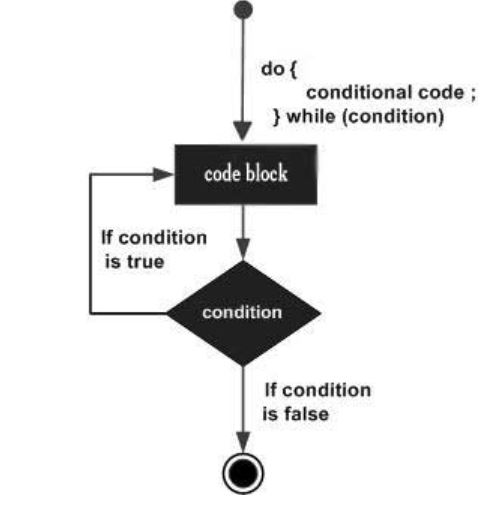×

Search anything:

# do while loop in C++

#### C++ Software Engineering do while loop loopGet this book -> Problems on Array: For Interviews and Competitive Programming

Loop is a programming concept which is natively supported by nearly all programming languages. Loops are used to repeat a particular coding task where each repetition follows a particular pattern which can be incorporated in a code.

The concept of loops comes into picture when we need to implement/print a particular block/statement more than one time till a particluar condition is specified.
We have different types of loops :

Entry Controlled loop : Where the entry of the loop is controlled that is we have a condition at the starting of the loop.
Example : while loop

Exit Controlled loop : In this type of loop the exit from the loop is controlled that is we have a condition at the end of the loop.
Example : do..while loop

The do...while loop is a variant of the while loop with one important difference. The body of do...while loop is executed once before the test expression is checked.

Syntax :

``````do
{
// codes;
}
while (testExpression);
``````

### How do...while loop works

1. The codes inside the body of loop is executed at least once. Then, only the test expression is checked.

2. If the test expression is true, the body of loop is executed. This process continues until the test expression becomes false.

3. When the test expression is false, do...while loop is terminated.### Difference between while and do...while loop

1. In while loop the controlling condition appears at the start of the loop.But in d...while loop the controlling condition appears at the end of the loop.

2. In while the iterations do not occur if, the condition at the first iteration, appears false but in do...while it occurs at least once even if the condition is false at the first iteration.

### Example

``````#include <iostream>
using namespace std;

int main ()
{
// Local variable declaration:
int a = 10;

// do loop execution
do
{
cout << "value of a: " << a << endl;
a = a + 1;
} while( a < 20 );

return 0;
}
``````

Output :

``````value of a: 10
value of a: 11
value of a: 12
value of a: 13
value of a: 14
value of a: 15
value of a: 16
value of a: 17
value of a: 18
value of a: 19
``````

### Question

Consider the following code:

``````#include <stdio.h>
int main()
{
int c = 5, no = 10;
do {
no /= c;
} while(c--);

cout<<no;
return 0;
}
``````

#### What will be the output of the above C++ program?

Run time error
1
0
Compile time error
There is a bug in the above program. It goes inside the do-while loop for c = 0 also. So it fails during runtime.
Read about for loop in C++: an alternative to do while loop#### Harshita Sahai

Maintainer at OpenGenus | Previously Software Developer, Intern at OpenGenus (June to August 2019) | B.Tech in Information Technology from Guru Gobind Singh Indraprastha University (2017 to 2021)

do while loop in C++